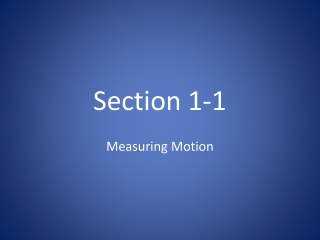Download PresentationSection 1-1

# Section 1-1

Télécharger la présentation## Section 1-1

- - - - - - - - - - - - - - - - - - - - - - - - - - - E N D - - - - - - - - - - - - - - - - - - - - - - - - - - -
##### Presentation Transcript

1. Section 1-1 Measuring Motion

2. Reference Point • Define: An object that appears to stay in place • Examples: the Earth, trees, buildings, etc.

3. Motion • Define: An object’s change in position relative to a reference point • How can you describe: With a reference direction (north, south, …)

4. Speed Depends on Distance and Time • Speed is: the distance/ the time • Standard (SI) unit is: meters/ second • Average speed • Most of the time objects don’t travel at a constant speed • Average speed = total distance/ total time

5. Speed graph

6. Practice Problems • What is the average speed if you take 0.5 hours to jog 4,000m?

7. Answer • Avg speed = total distance/ total time • = 4,000m/ 0.5 hours • = 8,000 m/hr

8. Practice problem #2 • If average speed is 110 km/hr, how long will it take the car to travel 770 km?

9. Answer • Time = distance/ speed • = 770 km/ 110 km/hr • = 7 hrs

10. Velocity • Speed and velocity DO NOT have the same meaning • Define: The speed of an object in a particular direction • Examples: 600 km/hr south, 10 ft/ sec northwest

11. Changing velocity • Think of velocity as: the rate of change of an object’s position • Velocity is only constant if both: speed and direction don’t change

12. Combining velocities • Velocities can be ADDED or SUBTRACTED depending on the direction • Resultant velocity: combination of two or more velocities

13. Same direction

14. Opposite direction

15. Acceleration • The rate at which velocity changes (Speed, direction, or both can change) • Positive acceleration = an increase in velocity • Negative acceleration or deceleration = a decrease in velocity

16. Acceleration continued • Units = meters per second per second • Equation: Final velocity- initial velocity Final time – initial time

17. Acceleration Graph Positive acceleration Negative acceleration or Deceleration

18. Circular Motion • An object traveling in circular motion is always changing its direction • Therefore, velocity/ acceleration is always changing

19. Circular motion drawing 5 m/s north 5 m/s south 5 m/s east 5 m/s west